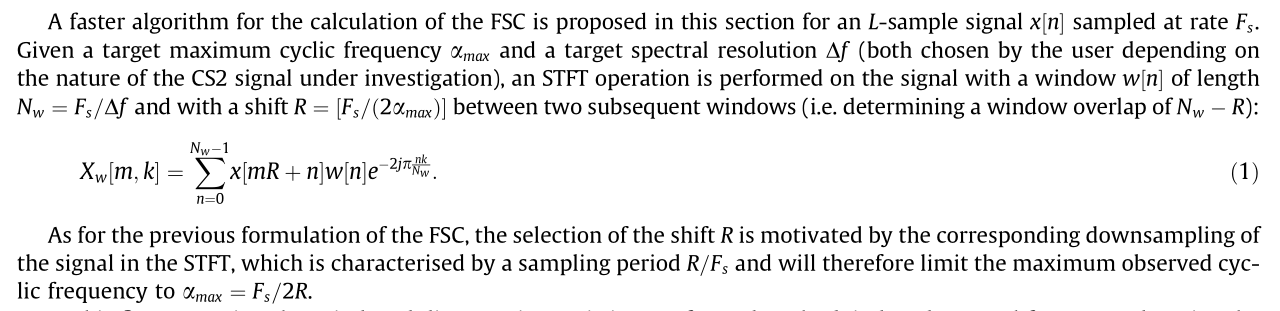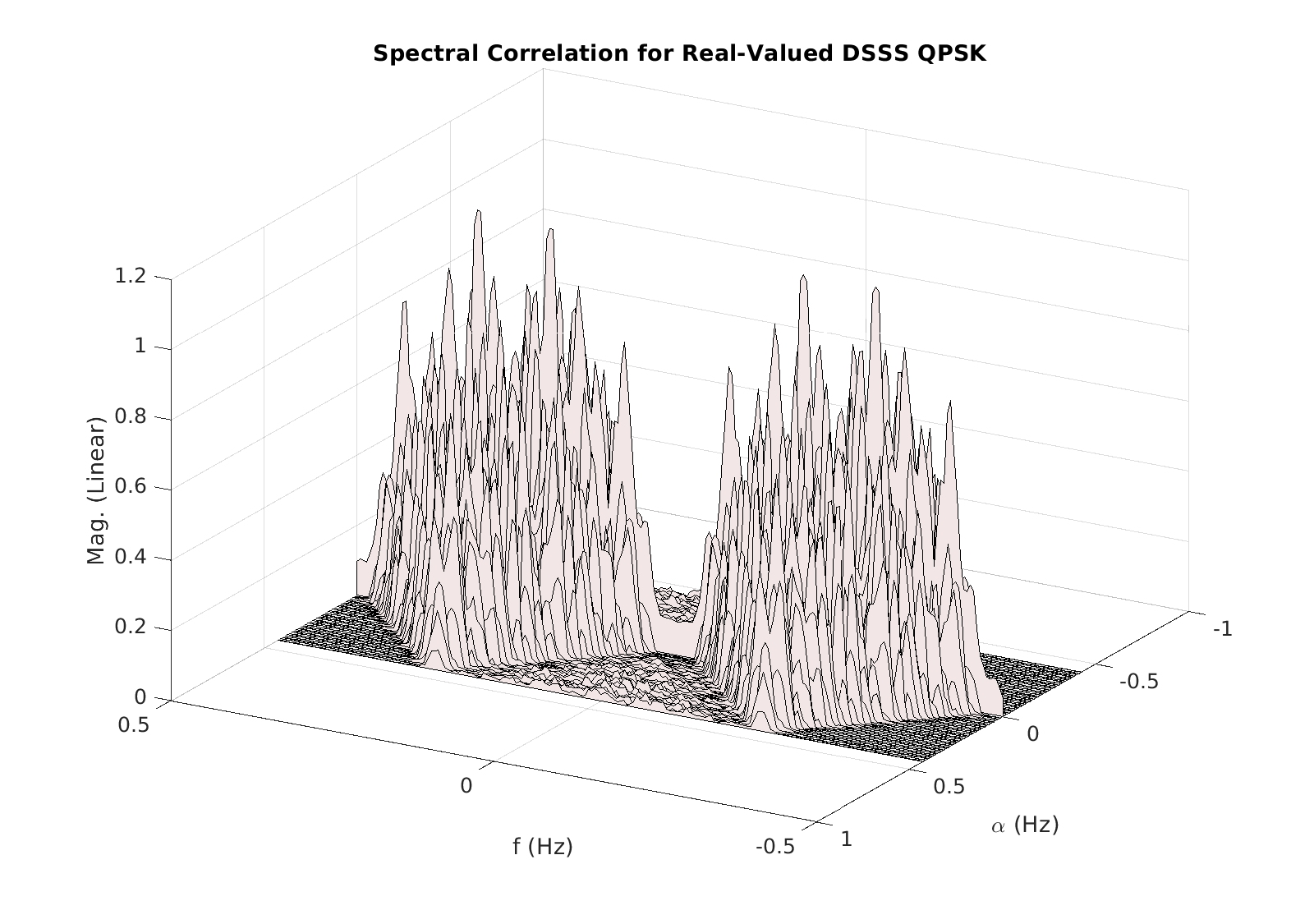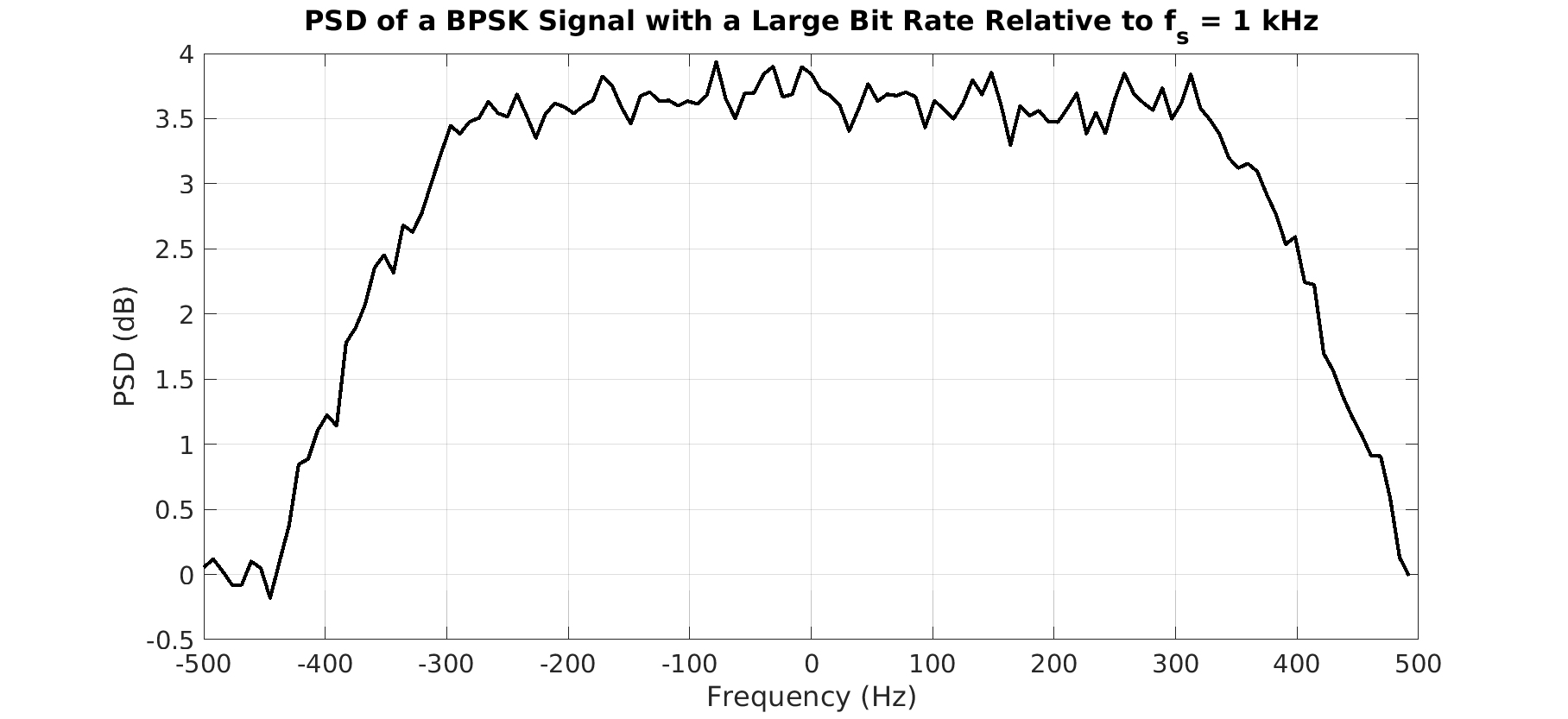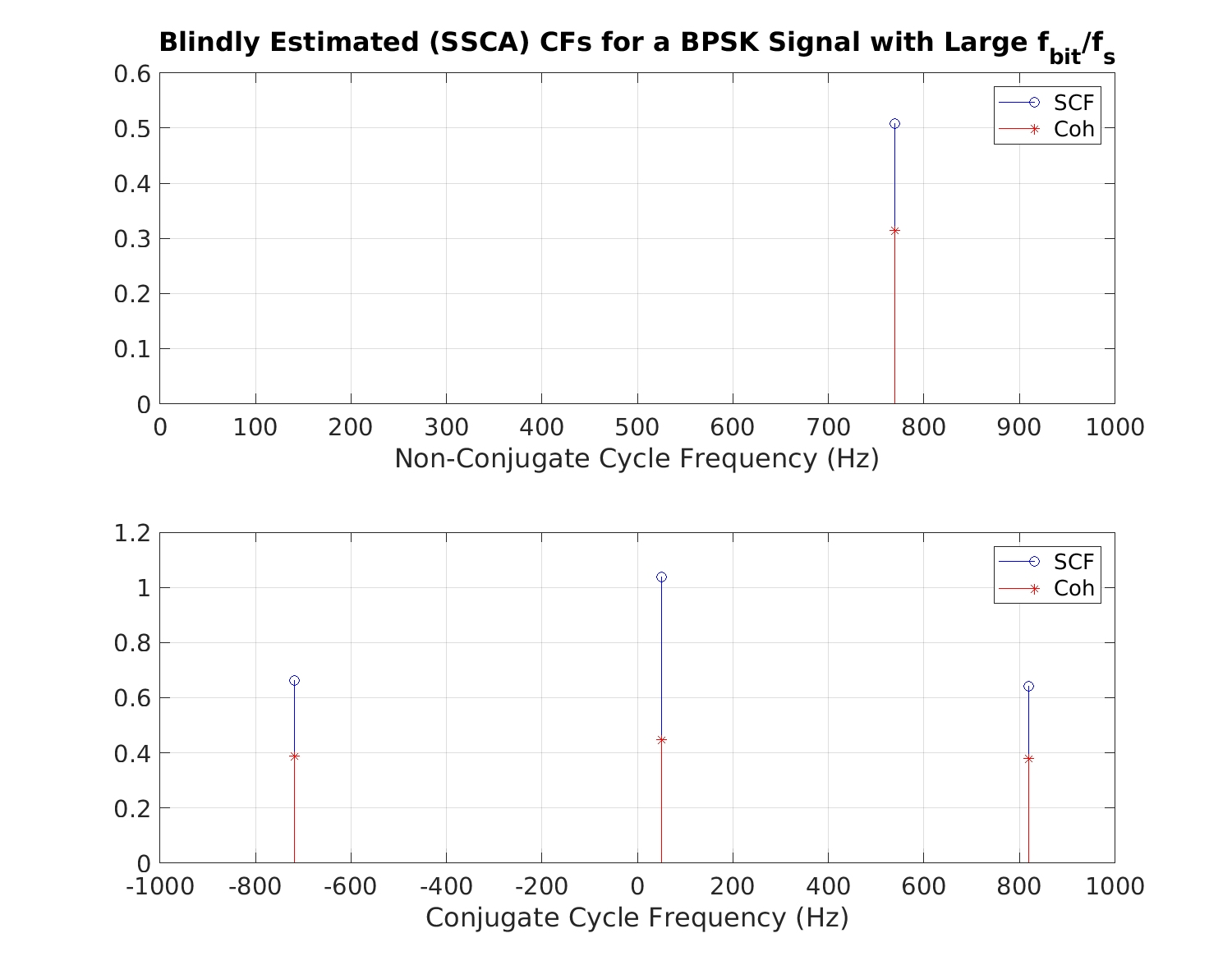# Update on J. Antoni’s Fast Spectral Correlation Estimator

Let’s take a look at an even faster spectral correlation function estimator. How useful is it for CSP applications in communications?

Reader Gideon pointed out that Antoni had published a paper a year after the paper that I considered in my first Antoni post. This newer paper, The Literature [R172], promises a faster fast spectral correlation estimator, and it delivers on that according to the analysis in the paper. However, I think the faster fast spectral correlation estimator is just as limited as the slower fast spectral correlation estimator when considered in the context of communication-signal processing.

And, to be fair, Antoni doesn’t often consider the context of communication-signal processing. His favored application is fault detection in mechanical systems with rotating parts. But I still don’t think the way he compares his fast and faster estimators to conventional estimators is fair. The reason is that his estimators are both severely limited in the maximum cycle frequency that can be processed, relative to the maximum cycle frequency that is possible.

Let’s take a look.

### What the Paper Says

The authors take a closer look at their previous short-time Fourier-transform method of computing the spectral correlation function for small values of the cycle frequency, and replace some of the operations with faster ones.

Antoni starts off describing why this is worth doing: traditional methods of spectral correlation estimation are too slow. The core of the argument is in Figure 1.Figure 1. The explanation in The Literature [R172] for why the averaged cyclic periodogram (ACP) is so costly. However, neither of the ACPs presented and used on the CSP Blog, and in the CSP literature known to me use this method.

Antoni attributes the bulk of the slowness of the time-smoothing method to the multiplication of the input data by complex exponentials with frequencies$\alpha \pm 2$ (or just one with value of$\alpha$). But that multiplication is never necessary in the time-smoothing method (TSM), which is an ACP, or in the frequency-smoothing method, which is also an ACP.

The real problem with those methods is the computation of the cyclic periodogram for each cycle frequency of interest; see the computational costs post for more details.

The authors then go on to discuss the approach to their method, and they explicitly state that the algorithm user must choose a maximum cycle frequency$\alpha_{max}$, as first mentioned in Figure 2.Figure 2. In setting up the algorithm development, the authors explicitly state that the largest possible value of the cycle frequency is half the sampling rate divided by the stride parameter . Note that has a minimum value of unity, so that the largest possible value for is half the sampling rate.

This is strange, because we know that the cycle frequencies in discrete-time CSP range from$-1$ to$+1$.

But it isn’t a misreading. Later we see that the authors equate a full spectral correlation analysis to looking at all cycle frequencies up to half the sampling rate, as seen in Figure 3.Figure 3. Equating a full cyclic spectral analysis (spectral correlation analysis) to examining the spectral correlation function for cycle frequencies from zero to half the sampling rate.

After the brief explanation of the new, faster version of the limited-cycle-frequency estimator, Table 3 shows how much faster the new method is relative to the old method, and throws in some computational counts for the “traditional operations.” But I can’t figure out if that means his idea of the ACP (TSM) or the strip spectral correlation analyzer (SSCA), which is also mentioned in the paper.Figure 4. Antoni’s comparison of the computational costs for the old and new versions of his algorithm.

What is important to note is the fourth column. The computational advantage is largest for the largest considered$\alpha_{max}$, which is$13\%$ of half the sampling rate, or about$6%$ of the sampling rate. In other words, for a spectral correlation analysis that looks only at$1/20$ of the possible cycle frequencies exhibited by the data.

Whether “Complexity traditional Operations” refers to Antoni’s version of the TSM, my version of the TSM, or the SSCA, the comparison is inapt if we take seriously the idea that only very small cycle frequencies are of interest. We’ll discuss that more below.

### The Maximum Possible Cycle Frequency is Not Half the Sampling Rate

Whether the data to be processed is real-valued or complex-valued, the maximum value of the cycle frequency is the sampling rate. When we operate in normalized frequencies, as we often do here at the CSP Blog, then the cycle frequency ranges from$-1$ to$+1$ for both the non-conjugate and conjugate spectral correlation functions. However, the non-conjugate spectral correlation function for$\alpha < 0$ is redundant with that for$\alpha > 0$; the non-conjugate spectral correlation function has some strong symmetries, so we can always ignore$\alpha < 0$. For the conjugate spectral correlation, there is no such symmetry, so we generally have to look over all of the interval$[-1, 1]$.

But Antoni et al are interested in real-valued signals, so there is no distinction between the non-conjugate and conjugate spectral correlation functions. They are identical. However, we still need to consider all cycle frequencies on$[0, 1]$ in the general case. Especially for processing communication signals. And the tagline or motto or subtitle or raison d’etre or whatever for the CSP Blog is “Using and Understanding the Statistics of Communication Signals,” so that’s what I will continue to focus on. (Also … the people that originally brought The Literature [R152] to my attention are also focused on communication signals, so I need to stay on topic for them too.)

Using real-valued signals doesn’t imply that the maximum cycle frequency that can be exhibited by some data is half the sampling rate. Let me give a couple numerical examples to illustrate and validate the idea.

First consider a real-valued BPSK signal with a carrier frequency near half the sampling rate. A PSD estimate for such a simulated signal is shown in Figure 5.

We expect this signal to have cycle frequencies equal to plus-and-minus the bit rate, the doubled-carrier plus-and-minus the bit rate, and the negative doubled-carrier plus-and-minus the bit rate. And zero. Don’t forget about power. And that is what we see when we pass this data through the FFT Accumulation Method (FAM), the SSCA, the TSM-in-a-loop, or the FSM-in-a-loop. Figure 6 shows the spectral correlation surface for blindly estimated cycle frequencies (SSCA) followed by high-fidelity spectral correlation function estimation for just those cycle frequencies (FSM).Figure 6. The spectral correlation magnitude surface for the simulated real-valued BPSK signal in Figure 5. Note that several of the important cycle frequencies are greatly in excess of half the sampling rate (0.5).

We can also see important cycle frequencies in excess of half the sampling rate for signals whose carrier is not pushed near half the sampling rate, such as for the real-valued direct-sequence spread-spectrum QPSK signal with PSD shown in Figure 7.

Using the same SSCA-based blind-CF estimation step followed by high-fidelity spectral correlation function estimation by the FSM, we obtain the surface shown in Figure 8.Figure 8. Spectral correlation surface for a real-valued simulated DSSS QPSK signal. Note that the significant cycle frequencies extend to nearly half the sampling rate ().

In the previous post, I also showed an example of a complex-valued communication signal with a single non-zero non-conjugate cycle frequency that was greatly in excess of half the sampling rate: BPSK with square-root raised-cosine pulses that is nearly critically sampled. That example is recreated in Figures 9 and 10 here.Figure 9. PSD estimate for a complex-valued BPSK signal that has been sampled at very nearly its Nyquist rate.Figure 10. Blindly estimated cycle frequencies for the critically sampled BPSK signal in Figure 9. Notice that three of the four cycle frequencies have values in excess of half the sampling rate of kHz.

### A Better Comparison

The comparison in Table 3 (Figure 4 here) to “traditional” estimators is not particularly apt. The reason is that if you want to focus exclusively on tiny cycle frequencies (and sometimes I do! Tunneling!), then you can often drastically bandlimit your data, subsample (decimate), and process the new data sequence. The overall number of seconds of processing-block length is the same as you started with, but the number of samples is much smaller, and so is the range of cycle frequencies that can be estimated.

For instance, if we take the signal Antoni bundles with his code seriously, we see that it is oversampled, as in Figure 11 (which is Figure 3 in the original post).

Further, we see that the cycle frequencies are plus-and-minus$10$ Hz. The features are centered at the signal’s apparent center frequency of$200$ Hz (see Figure 12). So one may filter around the obvious peak, shift to zero, decimate, and then perform an exhaustive spectral correlation analysis of that data. And the cost of that exhaustive analysis will be much smaller than the exhaustive analysis applied to the oversampled signal, because the number of samples could be reduced by a factor of at least ten.

But the same trick doesn’t apply to Antoni’s method–it requires oversampled data. If you apply it to the reduced data as I outlined, then you’ll be stuck with an$\alpha_{max}$ that is a small fraction of the new sampling rate.

### Conclusion

So, yes, the new Antoni algorithm is faster than the old Antoni algorithm. They are both still of very limited value in the context of communication-signal processing due to the highly constrained maximum cycle frequency that can be accommodated by the method.

Looking at Table 1 in the previous post, we can say that the new algorithm narrows the gap between the faster ‘SSCA for all cycle frequencies’ and the slower ‘FSC for a small fraction of cycle frequencies.’ But the new paper does not consider that outside of the FSC framework, we can simply refuse to oversample the signal. Then the gap widens, again, between ‘SSCA for all cycle frequencies’ and ‘FSC for a small fraction of cycle frequencies.’1.Gideon Genadi Kogan says: